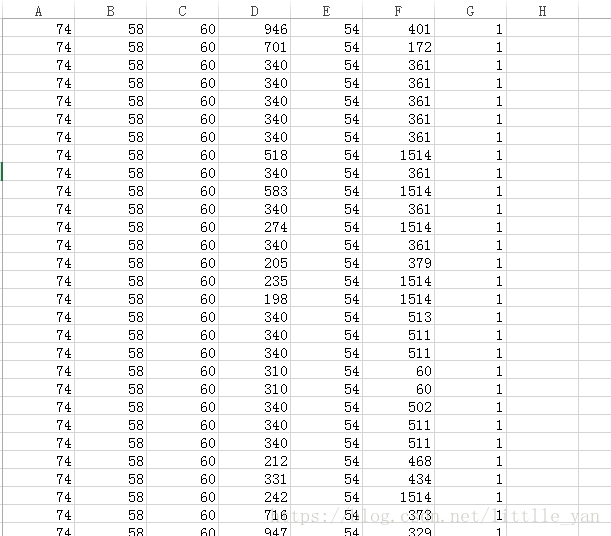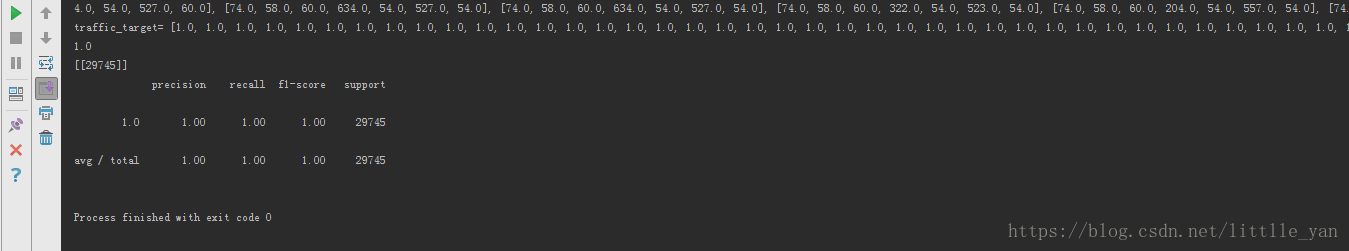基于sklearn实现Bagging算法（python）

更新时间：2019年07月11日 16:37:29   作者：little_yan_yan我要评论from sklearn.ensemble import BaggingClassifier
from sklearn.tree import DecisionTreeClassifier
from sklearn.preprocessing import StandardScaler
import csv
from sklearn.cross_validation import train_test_split
from sklearn.metrics import accuracy_score
from sklearn.metrics import confusion_matrix
from sklearn.metrics import classification_report
data=[]
traffic_feature=[]
traffic_target=[]
for content in csv_file:
content=list(map(float,content))
if len(content)!=0:
data.append(content)
traffic_feature.append(content[0:6])//存放数据集的特征
traffic_target.append(content[-1])//存放数据集的标签
print('data=',data)
print('traffic_feature=',traffic_feature)
print('traffic_target=',traffic_target)
scaler = StandardScaler() # 标准化转换
scaler.fit(traffic_feature) # 训练标准化对象
traffic_feature= scaler.transform(traffic_feature) # 转换数据集
feature_train, feature_test, target_train, target_test = train_test_split(traffic_feature, traffic_target, test_size=0.3,random_state=0)
tree=DecisionTreeClassifier(criterion='entropy', max_depth=None)
# n_estimators=500:生成500个决策树
clf = BaggingClassifier(base_estimator=tree, n_estimators=500, max_samples=1.0, max_features=1.0, bootstrap=True, bootstrap_features=False, n_jobs=1, random_state=1)
clf.fit(feature_train,target_train)
predict_results=clf.predict(feature_test)
print(accuracy_score(predict_results, target_test))
conf_mat = confusion_matrix(target_test, predict_results)
print(conf_mat)
print(classification_report(target_test, predict_results))